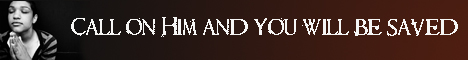Note:  Do not rely on this information. It is very old.

# Tangent

Tangent. A curve may be cut by a line in two or more points, and such a line is called a chord, but these points may be made to approach each other as the chord varies its position until at last they become coincident. When this occnrs, the line is known as a tangent to the curve. In the case of a circle or any other conic section, the tangent touches the curve at two consecutive points. these two points occur at A, the tangent there being the limiting case of the chord C D. In the case of a curve, such as that formed by the letter S, for example, which can be cut by a straight line in three points, the tangent may touch it at three coincident points and cut it at the same time; or it may touch it at two coincident points and cut it at a third. This passing of the tangent from one side of the curve to the other will occur whenever a chord cuts the curve in an uneven number of points; when, on the contrary, a chord cuts it in an even number of points, the tangent leaves the curve on the same side as it met it.

In trigonometry, the word tangent is used to denote a certain function of an angle. Thus, if B A C be any angle (1), and P M be drawn from any point P in A B perpendicular to A C, the ratio of the perpendicular P M to the base A M is known as the tangent of A. This is usnally abbreviated into P M / A M = tan A, The old definition of tangent, was somewhat different from this. Thus, if B C were the arc of a circle whose centre was A (2), and if T C were the tangent to the circle at C, meeting the radius A B in T, then T C was said to be the tangent of the arc B C. Since it was a line, and not a ratio, its value depended on the radius of the circle. The radius of the circ1e had therefore to be always given. The modern definition overcomes this difficulty, and the tangent of an angle has a perfectly constant value. In this case -

tan A is T C / A C = tangent of the arc / radius of the circle

which connects the values of the old and new functions.